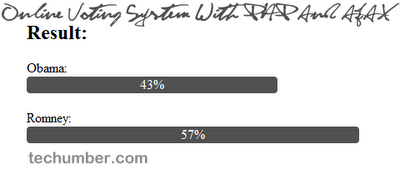# Online Voting System With PHP And AJAX

From last few days almost every day there is some news about Obama and Romney. Its been very interesting topic around the world that who will be the next president of USA. I thought this would be a great idea of creating an Online Voting System for next election(just for fun). Before you going to read the post check out the demo and vote you like..png “Online Voting System With PHP And AJAX:techumber.com”).png)

demo

Create following files.
–index.html
–vote.php
index.html

``````<div id="vote">
<form>
<input type="radio" name="vote" value="o" onclick="doVote(this.value)" />Obama
<br />
<input type="radio" name="vote" value="r" onclick="doVote(this.value)" />Romney
</form>
</div>

``````

This is a simple form with two radio buttons you can choose one. OnClick event will call the javascript function doVote().
dovote()

``````function doVote(v){
var xhr;
if (window.XMLHttpRequest){
xhr=new XMLHttpRequest();
}
else{
}
if (xhr.readyState==4 && xhr.status==200){
document.getElementById("vote").innerHTML=xhr.responseText;
}
}
xhr.open("GET","vote.php?v="+v,true);
xhr.send();
}

``````

In this function first we will create XMLHttpRequest Object. Show loading image until we get response.
When the response we will display the result.
vote.php

``````\$v = \$_GET['v'];
//Here our db will be txt file.
\$db = "result.txt";
//reads file conts to array
\$content = file(\$db);
\$arr = explode(":", \$content);
//obama and Romney counts
\$o = \$arr;
\$r = \$arr;
//new values updation
if (\$v== o){
\$o = \$o + 1;
}else{
\$r = \$r + 1;
}
//updateing new values to file
\$update = \$o.":".\$r;
\$fp = fopen(\$db,"w");
fputs(\$fp,\$update);
fclose(\$fp);
?>
<h2>Result:</h2>
<span>Obama:</span>
<p class="result" style="width:<?php echo(100*round(\$o/(\$r+\$o),2)); ?>%">
<?php echo(100*round(\$o/(\$r+\$o),2)); ?>%
</p>
<br />
<span>Romney:<span><p class="result" style="width:<?php echo(100*round(\$r/(\$r+\$o),2)); ?>%">
<?php echo(100*round(\$r/(\$r+\$o),2)); ?>%
</p>

``````

In this script we will capture the vote value. Update the new values to a file and send back the result in html format. All the votes values will be stored in result.txt.
That’s it! Hope you like it. Please comment my work.